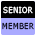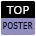Welcome to WebmasterWorld Guest from 18.232.171.18

Forum Moderators: open

# What are global variables?

#### Ron_Peters

11:37 am on Aug 13, 2019 (gmt 0)

#### New User from IN

joined:Aug 13, 2019
posts:2
votes: 0

How are these variable declared and what are the problems associated with using them?

#### NickMNS

4:07 pm on Aug 13, 2019 (gmt 0)

#### Senior Memberjoined:Apr 1, 2016
posts:2662
votes: 794

A Global is variable a that is declared outside of a function. The problem with using a Global variable is that it can be accessed and changed anywhere in the script, which can lead to unintended consequences.

Variables have a scope. A variable declared in a function, results in the scope of that variable being only with that function. So say you have a function "myFunc" and you declare a variable "x". You can assign a value to x, var x = 12. Then later in the function you can re-assign a value to it, say by incrementing it x =+ 1. The value of is then x == 13.

`` function myFunc() { var x = 12; if (some condition) { x += 1; } console.log('x is equal to:', x);}``

This is simple enough. Now let's add a function in myFunc call it innerFunc.
Here is a working demo: [jsfiddle.net...]
`` // define innerFuncfunction innerFunc(z) { var y = 1; if (z >= 12) { z += y; y += 1;  }}// define myFunc using innerFuncfunction myFunc() { var x = 12; innerFunc(x); console.log('x is equal to:', x); console.log('y is equal to:', y);}//call myFuncmyFunc();``

Now if you run this script you will see in the console that the value of x = 12 and that y throws an error as undefined. The reason is that x has it's scope in myFunc, and the value of x is passed to innerFunc by assigning it to z, so x is never incremented myFunc and thus does not change. Y is declared in innerFunc and thus when calling it in my myFunc returns undefined as its scope is limited to innerFunc.

Now let's throw in a few globals.
demo: [jsfiddle.net...]
``// delcare globalsvar x = 12;var y = 1;// define innerFuncfunction innerFunc() { if (x >= 12) { x += y; y += 1;  }}// define myFunc using innerFuncfunction myFunc() { innerFunc(); console.log('x is equal to:', x); console.log('y is equal to:', y);}//call myFuncmyFunc();``

Now if you run this script you will see in the console that the value of x = 13 and that y no longer throws an error its value is now 2, as expected . Since x and y are globals they can be called and assigned in any function, in this simple case it works in our favor, but in a more complex script this could lead to some strange bugs as these values can be changed when one doesn't expect it. Aslo, you may notice that in the first instance innerFunc(z) had a parameter z that was assigned when calling the function. In the second case, z was eliminated. Had z been left in place the value of x would have remained unchanged as x would have been assigned to z without impacting x.

It is easy to use Global variable as they are more intuitive but as scripts get more complex they can lead to problems. Below is the script that shows the way to get desired result without using the Global variables.
demo: [jsfiddle.net...]
``// define innerFuncfunction innerFunc(z,y) { if (z >= 12) { z += y; y += 1;  } return [z,y]}// define myFunc using innerFuncfunction myFunc() { var x = 12; var a; a = innerFunc(x,1); console.log('x is equal to:', a); console.log('y is equal to:', a); }//call myFuncmyFunc();``

Note: to see the result of the demo you have to open the browser console in developer tools